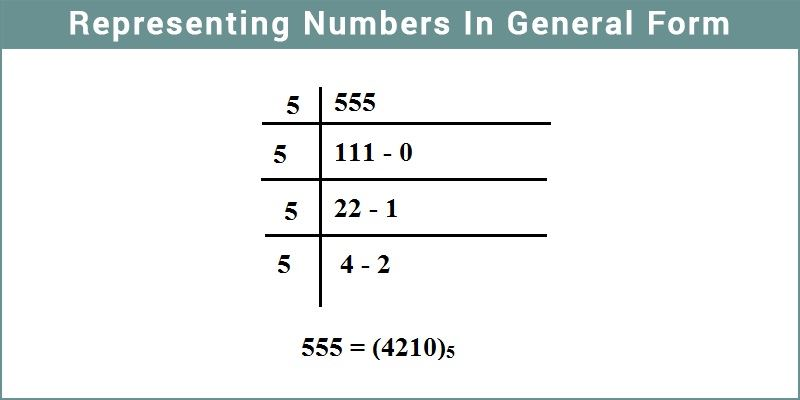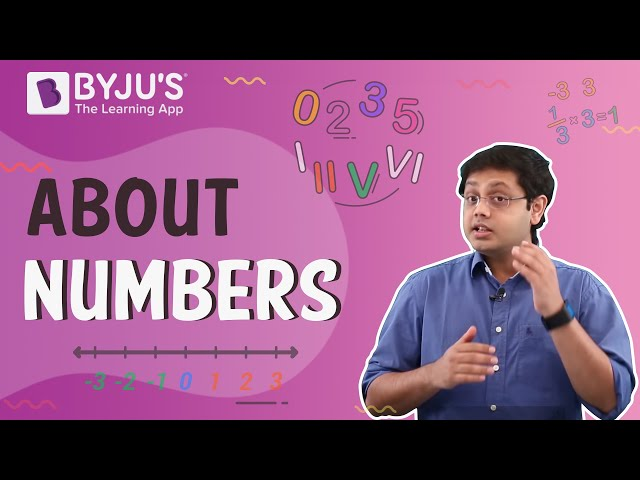# Representing Numbers In General Form

We come across numbers every day in our life. We buy a commodity with money and measure its quantity. Money and quantity all these are simply done with the help of numbers. Therefore the number plays a very significant role in our life. There are many types of numbers used in the number system based on convenience and requirements. For instance integers, whole and natural numbers etc which are often used for quantifying different things around us.A natural number is any positive number that is used generally. N = {1, 2, 3, 4, . . . . }

Whole numbers include all the natural numbers along with zero. W = {0, 1, 2, 3, 4, . . . . }.

An integer is any number which can be written without a fractional component. It belongs to a set of numbers that includes positive and negative numbers along with zero.

I = { . . . . . , -3, -2,-1, 0, 1, 2, 3, . . . }.

## Representation of Numbers in General Form

The general representation of a number is as follows:

For a two-digit number say AB = A × 10 + B × 1

For example, 95 = 90 + 5 = 9 × 10 + 5 × 1

For a three-digit number says ABC

= A × 100 + B × 10 + C × 1

= 100 A + 10 B + C

For example, 123 = 100 + 20 + 3

= 1 × 100 + 2 × 10 + 3 × 1

### Playing with Numbers

Numbers are quite amazing to play with and we can enjoy several tricks with them. We can reverse the digits and play. For example:

Steve and Mike sat down and Steve asked Mike to think of a two digit number. Mike did as he was told. Then Steve asked Mike to reverse the digits and add it to the original number. Then divide this number by 11. Mike followed. Steve concluded that after the division, there will be no remainder.

Let us suppose that Mike thought of the number 93. Reversing the digit we get 39. Adding them we obtain 132. Dividing by 11 we get the answer as 12. And there is no remainder. This happens because when we reverse the digits and add them, the number becomes a multiple of 11 and hence no remainder is left when the number is divided by 11:

10 a + b is the number taken.

Reversing the digits we get 10 b + a.

Adding them we get 11a + 11 b = 11 (a + b).

Therefore we obtain a number which is divisible by

$$\begin{array}{l}11\end{array}$$
.

## Video Lesson on Numbers